Next: References Up: Kinetic Formulations and Regularity Previous: Degenerate parabolic equations

# The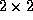isentropic equations

We consider thesystem of isentropic equations, governing the density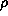and momentum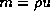,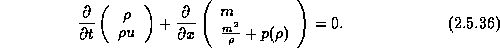Here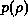is the pressure which is assumed to satisfy the (scaled)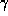law,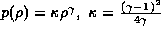.

The question of existence for this model, depending on the-law,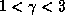, was already studied , by compensated compactness arguments. Here we revisit this problem with the kinetic formulation presented below which leads to existence result for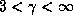, consult , and is complemented with a new existence proof for, consult .

For the derivation of our kinetic formulation of (2.5.36), we start by seeking all weak entropy inequalities associated with the isentropicsystem (2.5.36),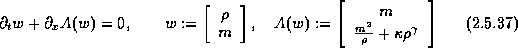The family of entropy functions associated with (2.5.37) consists of those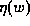's whose Hessians symmetrize the Jacobian, A'(w); the requirement of a symmetric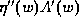yields the Euler-Poisson-Darboux equation, e.g,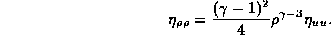Seeking weak entropy functions such that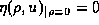, leads to the family of weak (entropy, entropy flux) pairs,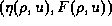, depending on an arbitrary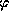,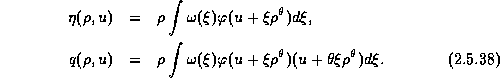Here,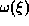is given by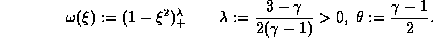We note thatis convex iffis. Thus by the formal change of variables,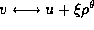, the weight functionbecomes the 'pseudo-Maxwellian',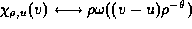,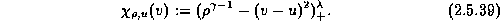We arrive at the kinetic formulation of (2.5.36) which reads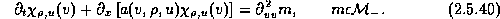Observe that integration of (2.5.40) against any convexrecovers all the weak entropy inequalities. Again, as in the scalar case, the nonpositive measure m on the right of (2.5.40), measures the loss of entropy which concentrates along shock discontinuities.

The transport equation (2.5.40) is not purely kinetic due to the dependence on the macroscopic velocity u (unless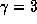corresponding to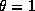),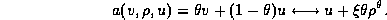Compensated compactness arguments presented in  yield the following compactness result.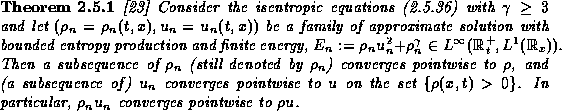Finally, we consider thesystem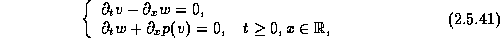endowed with the pressure law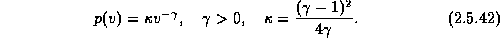The system (2.5.41)-(2.5.42) governs the isentropic gas dynamics written in Lagrangian coordinates. In general the equations (2.5.41)-(2.5.42) will be referred to as the p-system (see ,).

For a kinetic formulation, we first seek the (entropy,entropy flux) pairs,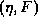, associated with (2.5.41)-(2.5.42). They are determined by the relations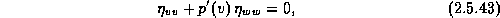where F is computed by the compatibility relations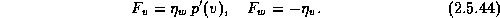The solutions of (2.5.43) can be expressed in terms of the fundamental solution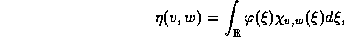where the fundamental solutions,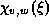, are given by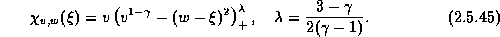Here and below,(rather than v occupied for the specific volume) denotes the kinetic variable. The corresponding kinetic fluxes are then given by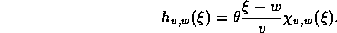We arrive at the kinetic formulation of (2.5.41)-(2.5.42) which reads,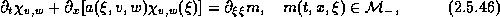with macroscopic velocity,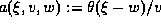.Next: References Up: Kinetic Formulations and Regularity Previous: Degenerate parabolic equations